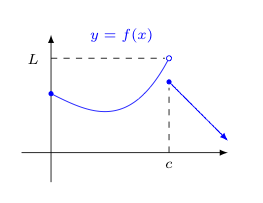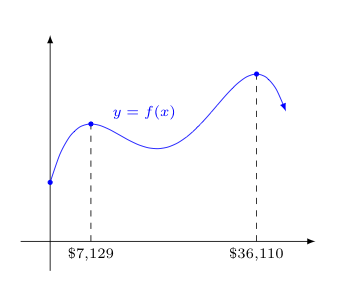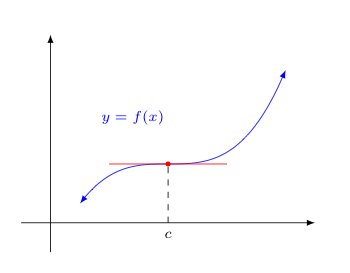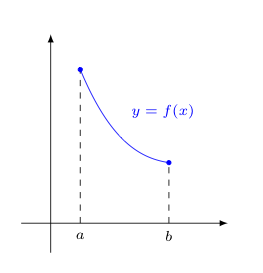## Extrema

One question often asked concerning a function is "How big (or small) will this function get?"

On one level, this question can be answered by establishing "bounds" on $f$. That is to say, it is helpful to at least know that there is some value $f(x)$ does not exceed (or fall below) -- or to know that no such value exists.

However, not all bounds are created equal -- some do a better job at "bounding" than others. For example, $-100 \le \sin(x) \le 100$ is certainly true -- but knowing that $-1 \le \sin(x) \le 1$ is likely to be far more helpful!

Interestingly, we can prove (using the epsilon-delta definition of limits and the definition of continuity) that any function that is continuous on a closed interval $[a,b]$ must be bounded (above and below) on that interval. This is known as the Boundedness Theorem.

Of course, we could give a better answer to the question above by actually finding the maximum (or minimum) value $f(x)$ attains, if there is one.

Notice, such a value need not always exist -- even if the function in question is bounded. For example, the following function is bounded above, but does not attain its maximum value due to the presence of a "hole on the left" associated with the gap discontinuity at $x=c$; nor does the function shown attain a minimum value, presuming the decrease shown on the right continues linearly.Now consider the following scenario -- suppose the function below gives the likely profit to you associated with an investment of $x$ dollars into some project. You are currently investing $\$7{,}129$, and are wondering if you should increase or decrease your investment. Unless you can substantially increase your investment, you are better off sticking with your current investment. As can be seen, any small change, either an increase or decrease, is going to cost you money! However, suppose you are able to invest anywhere from$\$0$ to $\$40{,}000$in this project. In that case, you should invest$\$36{,}110$ -- there is absolutely no other investment amount that would make you more money.This motivates the following definitions:

• A function $f$ has a local maximum at $c$, if and only if there exists an open interval containing $c$, on which $f$ is defined, such that $f(c) \ge f(x)$ for all $x$ in that interval.

• A function $f$ has a local minimum at $c$ if and only if there exists an open interval containing $c$, on which $f$ is defined, such that $f(c) \le f(x)$ for all $x$ in that interval.

In general, the above are called local extrema, as contrasted with what we now define below:

• A function $f$ whose domain contains some interval $I$ has an absolute maximum on the interval I at $c$, if and only $c$ is in this interval $I$ and $f(c) \ge f(x)$ for all $x$ in this interval.

• A function $f$ whose domain contains some interval $I$ has an absolute minimum on the interval I at $c$, if and only $c$ is in this interval $I$ and $f(c) \le f(x)$ for all $x$ in this interval.

These are in turn called, not surprisingly, absolute extrema.

If we seek to know if and where such extrema occur (both local and absolute), we should be aware of the following two important results. The first gives us a sufficient (but not necessary) condition for a function to have an absolute maximum and minimum. The second narrows the places we need to look, as we search for any local or absolute extrema present:

The Extreme Value Theorem
If $f$ is continuous on $[a,b]$, then $f$ has an absolute maximum and an absolute minimum in $[a,b]$.

Fermat's Theorem
If $f$ is differentiable at $c$ and $f(c)$ is a local extremum, then $f'(c)=0$.

Consider carefully how these can be used in conjunction with one another to find all of the extrema. For a given function $f$, there are likely only a few places (if any) where $f'(c)=0$. Importantly, Fermat's Theorem does not guarantee that finding these places will produce the extrema we seek (as the picture below demonstrates) -- but it allows us to eliminate a great number of places we might otherwise look.Of course, Fermat's Theorem doesn't have anything at all to say about extreme values where $f$ is not differentiable, so these represent additional critical places to look in our search for extrema.

With this in mind, we make the following definition:

Let $f$ be a function whose domain contains the value $c$. We say $c$ is a critical value if either $f'(c)=0$ or if $f$ is not differentiable at $c$.

So to find a function's extrema, we should definitely check the behavior of the function at all of its critical values.

Of course, if we are restricting our attention to just finding the extrema of a function $f$ on a given interval $[a,b]$, we'll need to consider what happens at the two ends of that interval as well, as the following picture suggests.Thus, to find the absolute minimum and absolute maximum of a function $f$ on an interval $[a,b]$, one should follow the following steps:

1. Find the function values at the critical values of $f$ on $[a,b]$
2. Find the function values $f(a)$ and $f(b)$
3. Identify the largest and smallest of the values found above. The largest value is the absolute maximum and the smallest is the absolute minimum.